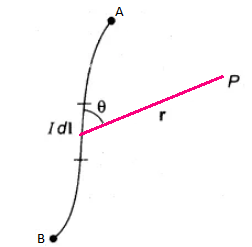# NEET MCQ on Biot Savart Law

This law states that the magnetic field (dB) at point P due to small current element Idl of the current-carrying conductor is directly proportional to the Idl (current) element of the conductor

dB ∝ IdlThe magnitude of the magnetic field is given by 丨dB丨= (μ0/4π)(IdIsinθ/r2)

Q1: Which is the equation of the magnetic field according to Biot-Savart’s law?

1. IdIsinθ/r2
2. 0/4π)IdIsinθ/r
3. 0/4π)IdIsinθ/r2
4. 0/4π)IdIsinθ

Q2: The magnetic field due to a copper rod carrying direct current is

1. The magnetic field is inside the rod
2. The magnetic field is outside the rod
3. Both inside and outside the rod
4. Neither inside nor outside

Answers: (c) Both inside and outside the rod

Q3: Consider a current i flowing through the inner conductor of a coaxial cable and the current returns along the outer conductor of the cable. The value of magnetic induction at a distance r from the axis is

1. 0/4π)(2i/r)
2. Zero
3. 0/4π)(2πi/r)

Q4: To which law of electricity is Biot Savart law of magnetism analogous to

1. Coulomb’s law
2. Ohm’s law
3. Kirchoff’s law

Q5: Which of the following properties cannot be calculated from Biot Savart law?

1. Magnetic field intensity
2. Flux density
3. Permeability
4. Electric field intensity

Q6: Consider a circular conductor with 2m radius and 8 A current flowing through it. What is the value of magnetic field intensity at the centre of the circular conductor?

1. 4π x 10-7 T
2. 8π x 10-7 T
3. 8 x 10-7 T
4. 3π x 107 T

Answers: (b) 8π x 10-7 T

Q7: If the strength of the magnetic field at a point r near a long straight current-carrying wire is B. The value of the field at a distance r/2 will be

1. B/2
2. B/4
3. 2B
4. 4B

Q8: If the current I flows through the coil of radius r then the field at the centre of the circular coil is

1. Inversely proportional to I2
2. Directly proportional to I
3. Directly proportional to r
4. Inversely proportional to r2

Answers: (b) Directly proportional to I

Q9: Two parallel conductors separated by a distance 2r carries current i in the same direction. The intensity of the magnetic field midway between them is

1. Zero
2. μ0 i/r
3. 0 i/r
4. μ0 i/4r

Q10: A straight wire of length 2 metres is taken and a current of 1 Ampere is passed through them. The magnetic field at a point along the axis of the wire at a distance of 3 metres from either end of the wire is given by

1. μ0 /4π
2. Zero
3. μ0
4. 0